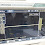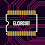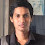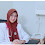# Super Power Amplifier Yiroshi Audio - 1000 Watt

This Yiroshi audio power amplifier has a low character and has high power output, so many audio lovers choose this type of amplifier because the bass boom is very pronounced. To make this Yiroshi audio amplifier so powerful need a power supply which is sufficient to let the results that can at maximum transformer minimum 20A 45-75v output and the good quality of components.

Below the circuit diagram of Yiroshi audio power amplifier driver + final transistors:
Component List
Resistor
R1=2k2, R2=47k, R3=100R, R4=100R, R5=56R, R6=56R, R7=56R, R8=56R, R9=47k, R10=100R, R11=2k2, R12=2k2, R13=100R, R14=47K, R15=2k2, R16=2k2, R17=100R, R18=100R, R19=100R, R20=100R, R21=220R/1W, R22=220R/1W, R23=100R, R24=1k8, R25=1k, R26=10k, R27=56R, R28=56R, R29=100R/1W, R30=100R/1W, R31=120R/1W, R32=120R/1W, R33=100R, R34=100R, R35,R36=220R/1W, R337,R38=220R/1W, R39,R40,R41,R42,R43,R44,R45,R53,R54,R56,R57,R58,R59=0R22/5W, R46,R47,R48,R49,R50,R1,R52,R60,R61,R62,R63,R64,R65,R66=10R/0.5W, R67=10R/5W + L1 10uH, R68=10R/1W

Capacitor
C1=1u Nonpolar, C2=330pF, C3=4n7, C4=4n7, C5=100uF/100V, C6=100uF/100V, C7=330uF/50V, C8=12pF, C9=12pF, C10=100N, C11=47pF, C12=150pF, C13=150pF, C14=100N

Transistor
Q1,Q4,Q7,Q8=2N5401
Q2,Q3,Q5,Q6=2N5551
Q9,Q13=2SC4793
Q10,Q12=2SA1837
Q11=MJE340
Q14=TIP2955/2SA1962
Q15=TIP3055/2SC5242
Q16,Q17,Q18,Q19,Q20,Q21,Q22=2SA1943 or you can change other PNP final power transistor
Q23,Q24,Q25,Q26,Q27,Q28,Q29=2SC5200 or you can change other NPN final power transistor

Final Power Transistor Audio Amplifier List
2SC5200/2SA1943 : 230volt 15 ampere 150 watt
2N3055/MJ2955 : 100 volt 15 ampere 150watt
TIP3055/TIP2955 : 100 volt 15 ampere 150watt
2N3773/2N6609 : 160volt 16 ampere 160watt
MJ15003/04 : 140volt 20 ampere 240watt
MJ15024/25 : 400volt 16 ampere 240watt

Sanken 2SA1295-2SC3264 : 200volt 17 ampere 200watt
Sanken 2sa1494-2sc3858 : 200volt 17 ampere 200watt
Sanken 2sa1216-2sc2922 : 180volt 17 ampere 200watt

Toshiba 2SA1095/2SC2565 : 160volt 15 ampere 150watt
2SC5200/2SA1943 : 230volt 15 ampere 150 watt
2N3055/MJ2955 : 100 volt 15 ampere 150watt
TIP3055/TIP2955 : 100 volt 15 ampere 150watt
2N3773/2N6609 : 160volt 16 ampere 160watt
MJ15003/04 : 140volt 20 ampere 240watt
MJ15024/25 : 400volt 16 ampere 240watt

Sanken 2SA1295-2SC3264 : 200volt 17 ampere 200watt
Sanken 2sa1494-2sc3858 : 200volt 17 ampere 200watt
Sanken 2sa1216-2sc2922 : 180volt 17 ampere 200watt
Toshiba 2SA1095 / 2SC2565 : 160volt 15 ampere 150watt
2SA1103 / 2SC2578 : 100volt 7 ampere 70 watt
2SA1105 / 2SC2577 : 120volt 9 ampere 90watt
2SA1106 / 2SC2851 : 140volt 10 ampere 100watt
2SC2580 / 2SA1105 : 120volt 9 ampere 90watt

2SC2581 / 2SA1106 : 140volt  10 ampere 100watt

Buy these parts, go to Allchips.

PCB Layout Driver Power Amplifier Yiroshi Audio

PCB Parallel Final Power Transistor 7 Set Toshiba 2SC5200 2SA1943

Final Power Transistor Assembling 2SC5200 and 2SA1943

Wiring Diagram Driver Power Amplifier and Final Power Transistor

For this Yiroshi power amplifier project, I make a separate PCB driver with the final transistor, you can see on both picture PCB Layout above. The power amplifier is very good and high performance. You can upgrade again to add power amplifier by adding the final transistor and also replace it with more power, current, and stronger voltage. And also must be balanced with a larger power supply current and voltage. For more details see the full interesting video below:

## 96 comments for "Super Power Amplifier Yiroshi Audio - 1000 Watt"

1.This circuit have some problems after 4 to 5 min power transistor dead

1.no, please see at circuit diagram. There is no erorr, you must adjust bias voltage.

2.it can work normally not just a minute.

2.Nice projekt and video! :D

1.thanks dear...

3.Good amplifier and thanks for your video

1.thank you, thanks for visit

4.Thanks for video and project.

1.youre wellcome, and say thanks for visit

5.we are happy to comment here, and we await your komantar in our blog

6.Hello,
The amp as shown in schematics will NOT work. Q15 should be NPN type, prrobably TIP3055, although I think this is a bad choice as TIP3055/TIP2955 have Vce max of only 70V.
Cheers

1.Q14=TIP2955/2SA1962
Q15=TIP3055/2SC5242
you can choose these transistor

2.the power amplifier is work, you can see the video testing amplifier

7.I saw some other videos the same circuit but there is no trimpot or bias circuit

1.yes, the circuit is no trimpot bias setting.

8.But on the assembling video i saw there is bias tripot I'm confused, but anyway thanks a lot for sharing this powerful amp i will try to build very soon. Thanks a lot god bless..

1.try to use it cause the trimpot can adjust bias voltage if you using any finalt transistor type.

9.what are the sizes of the PCB?

1.see pcb size on elcircuit stereo yiroshi

10.hai i want pcb and components all so price please my email id: [email protected]

1.you can contact me sir.

11.how to adjust the trimpot in the amplifier driver

1.you can set output voltage until 0v.

12.Hi sir romadhon, I'm back after building this power amp it sounds nice even though i tested it on 65v 8.5A so not enough power supply. Im Wondering on the pnp final output power they are warmer than the npn output i have noticed the predriver 2SA1837 for pnp output is warmer also is this normal? Because still sounds good or maybe i will get problems in the future. Please help

1.You can change the emitter resistor by using 220R 1w. and also the npn transistor

13.hi how are you can you tell me how to download the PDF, thanks

1.the pdf file is in stereo yiroshi amp post

14.Sir can i use 90v symmetrical ?

1.yes im using 110V its normally. but you must have larger heatsink

15.Sir what voltage should I use for 12pairs 5200/2943 transistor output?

1.you can use up to 100v for more performance

16.Can I use IRFP240 and IRFP9240 for this project?

1.It can't if you use final mosfet. see class d d4k5 its bigger power than this amp. if you want mosfet class d amp

17.sir i am using with mjl21194,mjl21193 14 pair ? which use driver ..and volt ? ampere ?..

1.you can use this driver or yamaha PA-2400, 80 upto 100V is recomended try using 20A current transformer ar SMPS circuit.

18.Thank you sir

19.Nice amp sir but sir you use cliping indictor and protection ckt

20.If i use 8 transistor instead of 14 transistor in the final driver Will the amplifier still work?

21.what current rating should the L1 coil be

1.max 20A output

22.Is it possible to send me better quality PCB, and PCB size, and layout files, also schematic diagram please [email protected]

1.2.23.Where do I put the trimpot to regulate the OFFSET voltage?

24.can you tell me the base voltages of 1943 and 5200 mine is .1 and .4 mv

1.set the base voltage by adjusting trimpot

25.can you tell me the base voltages of 1943 and 5200 mine is .1 and .4 mv

26.Puedes pasar el pdf y las medidas exactas de la placa en que parte del diagrama va el trimpot

27.Hi sir,how to order the PCB and components parts of 1kwatt amp.

28.29.i can't see the sizes of PCB sir ?

1.its actual size print.

30.hey..I want to buy all the components of this amplifier.. how can I contact u.. my email is [email protected]

1.https://www.ebay.com.au/itm/123348339586

31.Sir if i want to put trimpot which should i put there ?ty

1.See the schematic

32.Sir can i use in the circuit 10 pairs of final transistors then the speaker out is? Watts and Witch transformer I can use please tell me sir

1.You can use upto 20A transformer

33.Can i use 55ac o 55acv 20 ampere it is ok sir

1.2.What are the size pcb driver

34.Its nice sound.what is the best vac in power amp i have 7 set of 2sc5200 and 7set of 2sa1943 what is the best amphers and Vac 45 something?

35.Hey ' i need purchase this amplifier subwoofer kit ...how purchase ??

1.https://www.ebay.com.au/itm/123348339586

2.Did you sell it?

3.Yes after I corrected the errors on PCB layout, and found a schematic that was correct, have sold over 350 so far, I also have the Crown XLS 6 fet as well, with corrected schematic and PCB, only sold 275 of the so far

36.there is a huge demand for these amplifiers, but a lot of errors, or cannot read schematic, and exaggerating the output wattage...people want the truth, when they purchase, that's what I am doing

1.its so good bro

37.hola amigo tengo un problema en las resistencias de 220 y 100 ohs se me esta calentando y se terminan quemando como corrijo ese problema

38.schematic diagram doesn't have diodes and ohms for R24 and R25 should be flip. can you update the schematic diagram with trimpot

39.tem o kit pra vender?

40.Please,send me this pdf thanks ([email protected])

41.This is where you get PCB, with all the errors fixed, as the layout from wahyu eko romadhom will only work for approx 3 mins then it destroys itself, here is link to PCB that WORKS https://www.ebay.com.au/itm/123561661498?var=&ssPageName=STRK:MESELX:IT&_trksid=p3984.m1555.l2649

42.Hola disen que ay que cambiar 2 resistensias las lateralrs de 4k7 por 47k

43.how many watts on base on resistors? .25 watts or .5 watts

R1=2k2, R2=47k, R3=100R, R4=100R, R5=56R, R6=56R, R7=56R, R8=56R, R9=47k, R10=100R, R11=2k2, R12=2k2, R13=100R, R14=47K, R15=2k2, R16=2k2, R17=100R, R18=100R, R19=100R, R20=100R,

44.Hi sir i just need to ask where to adjust dc offset of this amp i need to adjust to 0V dc there's only one trimpot but adjust only for the current bias.

45.Hi sir i just need to ask where to adjust dc offset of this amp i need to adjust to 0V dc there's only one trimpot but adjust only for the current bias.

46.Hi You give file Gerber in email : [email protected] :-s

47.How to make the 10 uh coil bro
Explain the guage of wire and how many turns wanted

48.Sir it working but humming problems and transistors hitting very quickly

49.In the drawing of squematic,the Q15 transistor isn't wrong?

50.Dor,
Can you please Tell us where tô find a correct esquematic whith ALL the erros fixed.
After few minuts It burns!
In the squematic there os no trim por, Q15 os misdrawed, André i real life ,some resistores must vê chances.
Thank You Very much!!!!!

51.do you have available pcb layout for project,.,can i buy?

52.Sir....Can you reply... What is the PCB size...???

53.In the squematic there os no trim por, Q15 os misdrawed, André i real life ,some resistores must vê chances. no, please see at circuit diagram. There is no erorr, you must adjust bias voltage.

54.can you send me Gerber files sir.

55.can i use TTC5200 in exchnge 2sc5200

56.por los comentarios y lo visto otro MAS QUE NO SIRVE CERO Y VAN DOS si es que funciona deberias contestar dudas no creo que te cueste mucho trabajo y tener mas atencion con tus seguidores

57.Sir. I make 1000w mono amplifire using this driverboard. I want to use subwoofers on it. What can i do for it?

58.Have a reference blog / idol blog. Important for a blogger to have a reference Take for example, I like a blogger on this site.

59.Have a reference blog / idol blog. Important for a blogger to have a reference Take for example, I like a blogger on this site.

60.do you have preamp of yiroshi amplifier ?

61.This comment has been removed by the author.

62.What exactly is the quiet current in the yiroshi amplifier?

63.this amp has a trap door, replace the components with the xls602

este amplificador tiene una trampita, reemplace los componentes por los de la xls602

64.